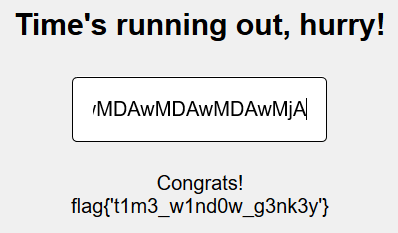HackPack CTF – Time Window Writeup

The evil server admin has a secret but will only give it to you if you can guess his coveted hidden value, but it keeps changing with time: https://timed-key.cha.hackpack.club

By analyzing the source of the web page we see that there are two JS files linked to it.

<script src="_sr_.js"></script>
<script src="__.js"></script>

However, when you submit the value, only the function c() is triggered.

<form onsubmit="javascript:c();return false;" action="javascript:c();">
<input type="text" id="message" autocomplete="off" autofocus />
</form>

c() function belongs to __.js file, so let’s analyze it more closely.

First, we need to format the code in a way easier to understand.

var x,
y,
dn = Date.now,
rc = function () {
return Math.floor(107 + 19 * Math.random())
},
rn = new Math.seedrandom('0x42').int32(),
rt = function () {
let r = Math.floor(1 + 7 * Math.random()),
e = [
];
for (; r > 0; ) e.push(rc()),
r--;
return String.fromCharCode(...e)
},
rr = function (r, e) {
for (; r > 0; ) e.unshift(e.pop()),
--r;
return e.join('')
},
lr = function (r, e) {
for (; r > 0; ) e.push(e.shift()),
--r;
return e.join('')
},
cf = function (r) {
try {
let e = Array.from(atob(rr(243, Array.from(r)))),
n = 1;
for (let r = 0; r < e.length; r++) r === n && (e.splice(r + 1, + e[r + 1] + 1), n += n);
return + lr(168, e)
} catch (r) {
}
return - 1
},
c = function () {
let r = document.getElementById('message').value,
e = document.getElementById('msg');
x = rn % 2 == 0 ? lr : rr,
y = rn % 2 != 0 ? lr : rr,
cf(r) >= dn() - 60000 ? fetch('/check', {
method: 'POST',
mode: 'same-origin',
cache: 'no-cache',
'Content-Type': 'application/json'
},
referrer: 'no-refferer',
body: JSON.stringify({
key: r
})
}).then(r=>r.json()).then(r=>{
r.hasOwnProperty('flag') ? e.innerHTML = 'Congrats! <br/>' + r.flag : e.innerText = 'Nope!!!'
}).catch (r=>{
console.error(r)
})  : e.innerText = 'Nope!!!'
};

By analyzing c() function, we see that if the inputted value is correct, a POST request is made and the flag is retrieved.

But how do we know if the value is correct?

For the request to happen the following statement should be true:

cf(r) >= dn() - 60000

dn was early defined:

dn = Date.now

So, dn() should return the current date and time in epoch format.

If you open your js browser console and write dn() in it, you should see a number like 1587643549542 as output.

Now, simply subtract 60000 from it (you can write dn() – 60000 in your console).

We now know that our input should return a number bigger than dn() – 60000 (for example, 160000000000) when passed into cf() function.

But what does cf() function do?

To understand what it does, we also should take a look at rr() and lr() functions.

rr(r,e) takes a number (r) and an array (e) as parameters, removing the last item of the array and adding it to the beginning r times, returning the resulting array as a string.

So, rr(2, [“a”,”b”,”c”,”d”,”e”]) would result in “deabc”

lr(r,e) does exactly the contrary. It takes a number (r) and an array (e) as parameters, removing the first item of the array and adding it to the end r times, returning the resulting array as a string.

So, lr(2, [“d”,”e”,”a”,”b”,”c”]) would result in “abcde”

Decryption

Back to our cf() function, we realize that the inputted value is being converted to an array of chars and passed to rr() where it will suffer 243 iterations.

Then the returned string will be base64 decoded using atob() function.

The next step is a for loop, where for r = 1,2,4,8,16,32,64… the following code will be executed:

e.splice(r + 1, + e[r + 1] + 1)

That means that at position r + 1 the following + e[r + 1] + 1 characters will be removed. So, even if the char at position e[r+1] is 0, there is still 0+1 character that is going to be removed.

After this step, the resulting array is passed to lr() and it will suffer 168 iterations.

Encryption

To make sure the returned value is bigger than dn() – 60000, we will try to encrypt the value 20000000000000.

Because lr(168, e) is the last decryption step, we should start by calling rr(168, e) to encrypt it, being e an array containing our value characters.

rr(168, Array.from("20000000000000"))

And this returns 20000000000000 (if you choose a number that returns itself the task will become easier).

Because e.splice(r + 1, + e[r + 1] + 1) will remove characters at positions 1+1, 2+1, 4+1 and 8+1, four characters at total (one at time because the only non-zero value is the first and for the rest, 0+1 = 1)

So, I added 4 zeros at the end of the string so that when this step occurs, the resulting string is 200000000000000000.

At this moment, our payload is 200000000000000000.

Now, we need to convert it to base64 using btoa() function:

btoa("200000000000000000")

The resulting string is MjAwMDAwMDAwMDAwMDAwMDAw

And now, we only need to execute:

lr(243, Array.from("MjAwMDAwMDAwMDAwMDAwMDAw"))

The returned string, our payload, is wMDAwMDAwMDAwMDAwMDAwMjA.

And now, we have the flag:This challenge was solved by my team, ducks0ci3ty.